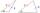Laws

From which law follows directly the validity of Pythagoras' theorem in the right triangle?

« Correct resultSolution:Leave us a comment of example and its solution (i.e. if it is still somewhat unclear...):Be the first to comment!To solve this example are needed these knowledge from mathematics:

Cosine rule uses trigonometric SAS triangle calculator. See also our trigonometric triangle calculator. Pythagorean theorem is the base for the right triangle calculator.

Next similar examples:

1. MedianThe median of the triangle LMN is away from vertex N 84 cm. Calculate the length of the median, which start at N.
2. Theorem proveWe want to prove the sentence: If the natural number n is divisible by six, then n is divisible by three. From what assumption we started?
3. Complex number coordinatesWhich coordinates show the location of -2+3iGiven that P = (5, 8) and Q = (6, 9), find the component form and magnitude of vector PQ.
5. Angles by cosine lawCalculate the size of the angles of the triangle ABC, if it is given by: a = 3 cm; b = 5 cm; c = 7 cm (use the sine and cosine theorem).
6. Isosceles IVIn an isosceles triangle ABC is |AC| = |BC| = 13 and |AB| = 10. Calculate the radius of the inscribed (r) and described (R) circle.
7. Triangle ABCIn a triangle ABC with the side BC of length 2 cm The middle point of AB. Points L and M split AC side into three equal lines. KLM is isosceles triangle with a right angle at the point K. Determine the lengths of the sides AB, AC triangle ABC.
8. Vector 7Given vector OA(12,16) and vector OB(4,1). Find vector AB and vector |A|.
9. Triangle - is RT?Triangle has a circumference of 90 cm. Side b is 1 cm longer than c, side c is 31 cm longer than side a. Calculate the length of sides and determine whether triangle is a right triangle.
10. Euclid2In right triangle ABC with right angle at C is given side a=27 and height v=12. Calculate the perimeter of the triangle.
11. Geometric progression 2There is geometric sequence with a1=5.7 and quotient q=-2.5. Calculate a17.
12. Two triangles SSATwo triangles can be formed with the given information. Use the Law of Sines to solve the triangles. A = 59°, a = 13, b = 14
13. Calculation of CNCalculate: ?
14. Greatest angleCalculate the greatest triangle angle with sides 197, 208, 299.
15. Side cIn △ABC a=2, b=4 and ∠C=100°. Calculate length of the side c.Find the roots of the quadratic equation: 3x2-4x + (-4) = 0.Prove whether you can construct a triangle ABC, if a=9 cm, b=10 cm, c=4 cm.